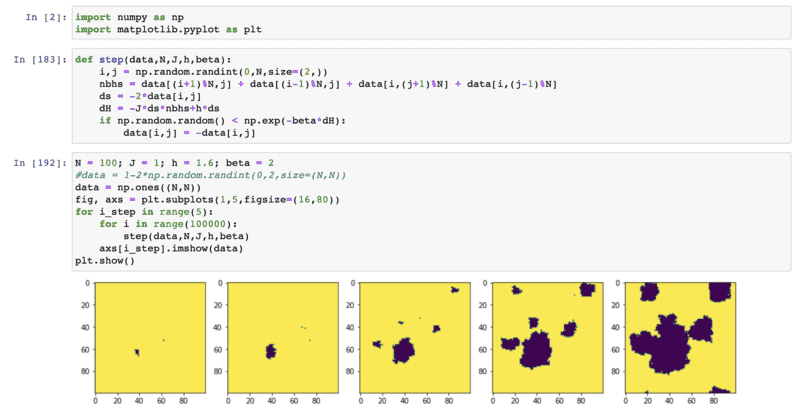# Bubble nucleation and metastable vacuum

• A
• paralleltransport

#### paralleltransport

TL;DR Summary
I'd like to have a better physical picture of metastable vacuum decay
Consider the decay in a quantum field theory from a metastable vacuum to the "true" vacuum.

Here's i my understanding:

1. For a scalar field potential U(\phi), the transition amplitude is finite per unit volume for a finite energy splitting between the 2 classical minima of the potential. This implies that there is a finite probability per time that some (finite region) subset of the spacetime decays into the true vacuum.

This transition amplitude can be computed by computing the action in euclidean time of a spherically symmetric instanton solution.

My question:

Why is this process called bubble nucleation? It is also said that it is related to phase transitions, but I fail to see the connection. Here one is talking about a tunneling amplitude to transition from one (false) vacuum to the real one.

Last edited:

I am not at all educated in QFT, so I read this wiki page (https://en.wikipedia.org/wiki/False_vacuum_decay).

Water can be found in the liquid phase even above 100 degrees C (at 1 atm). It is in a local minimum of the free energy so it is "relatively" stable. The thing is that, even if the gas phase is energetically favorable, it takes energy to create a bubble because you have to break water's surface tension to create a gas-liquid interface. This is the process of nucleation. It seems that when the "false" vacuum decays, the "true" vacuum appears in the systems like a bubble of water vapor in a bowl of water. So they just use all the know equations that were developed in the theory of nucleation to estimate stuff for this model. To me, it is just a matter of names, but I am not educated on this topic so I am just guessing.

The connection to phase transitions I guess is because of fluctuations. It seems to me that this metastable vacuum could decay due to quantum fluctuations. A similar thing happens in the liquid-vapor phase transition: the density of the liquid fluctuates in the system when you get close to the transition temperature and when the fluctuations are too big you will have that, in some small volume region, the density is low enough that a bubble of vapor forms.

•dlgoff and paralleltransport
Just to show some pictures, you can imagine the ising model for example - spins taking values in $\{+1,-1\}$, with a small ('longitudinal', I suppose) field biasing it so that $\{-1\}$ is the true vacuum. Explicitly, the hamiltonian looks something like:
$$H = -J\sum_{\langle i,j \rangle} \sigma_i \sigma_j + h\sum_i \sigma_i$$
where $\langle i,j \rangle$ is a sum over the neighboring pairs of spins. Then, the configuration of all $+1$ spins is still a local minimizer for $H$, but local fluctuations to $-1$ spin will grow and look like bubbles, for example showed in this plot•dRic2
Just to show some pictures, you can imagine the ising model for example - spins taking values in {+1,−1}{+1,−1}, with a small ('longitudinal', I suppose) field biasing it so that {−1}{−1} is the true vacuum. Explicitly, the hamiltonian looks something like:
H=−Ji,jσiσj+hiσiH=−J∑⟨i,j⟩σiσj+h∑iσi​
where i,j⟨i,j⟩ is a sum over the neighboring pairs of spins. Then, the configuration of all +1+1 spins is still a local minimizer for HH, but local fluctuations to −1−1 spin will grow and look like bubbles, for example showed in this plot
View attachment 296073

Very cool!

I think i understand now, thanks to you guys. The bubble in the quantum field theory case has a surface tension that is due to the domain wall (analog to surface tension of a 2-phase interface) while its volume is energy favored. A bubble above a certain critical value will be energetically favored and expand. Quantum mechanical tunnelling probability is the analog of statistical fluctuations which produce bubbles of various size, but only the one with large enough size will expand.